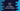# 3 Swift programs to find the largest of three numbers## Swift program to find the largest of three numbers:

In this post, we will learn how to find the largest of three user provided numbers. This program will ask the user to enter the numbers one by one, keep them in three variables and print out the largest of these three.

We can solve this in two different way. One way is to find out the largest value by comparing each values with one another.

We can also solve this by using the max method that gives the maximum value of two numbers.

Let’s try both of these methods one by one.

## Method 1: Find the maximum of three numbers by comparing the values:

Let’s try it by comparing the numbers with one another.

``````func findMax(x: Int, y: Int) -> Int{
return x > y ? x : y
}

func findMaxThree(first: Int, second: Int, third: Int) -> Int{
let maxFirstTwo = findMax(x: first, y: second)
let maxLastTwo = findMax(x: second, y: third)

return findMax(x: maxFirstTwo, y: maxLastTwo)
}

print(findMaxThree(first: 1, second: 2, third: 3))
print(findMaxThree(first: 1, second: 3, third: 2))
print(findMaxThree(first: 3, second: 1, third: 2))
print(findMaxThree(first: 3, second: 2, third: 1))
print(findMaxThree(first: 3, second: 3, third: 3))``````

For this program,

• findMax method is used to find the larger value between x and y.
• findMaxThree method is used to find the largest of three numbers. It finds the maximum of first and second numbers and keep that value in maxFirstTwo. Similarly the maximum of second and third is kept in maxLastTwo.
• Finally, it returns the maximum of maxFirstTwo and maxLastTwo variables.

### Output:

If you run this program, it will print the below output:

``````3
3
3
3
3``````

## Method 2: By using max() and two parameters:

We can also use the max() function to find the maximum of two numbers. We can remove findMax by max() as like below:

``````func findMaxThree(first: Int, second: Int, third: Int) -> Int{
let maxFirstTwo = max(first, second)
let maxLastTwo = max(second, third)

return max(maxFirstTwo, maxLastTwo)
}

print(findMaxThree(first: 1, second: 2, third: 3))
print(findMaxThree(first: 1, second: 3, third: 2))
print(findMaxThree(first: 3, second: 1, third: 2))
print(findMaxThree(first: 3, second: 2, third: 1))
print(findMaxThree(first: 3, second: 3, third: 3))``````

It will print the same output.

## Method 3: By using max() and three parameters:

We can also use max with three parameters. It will return the maximum value of three.

``````print(max(1, 2, 3))
print(max(1, 3, 2))
print(max(3, 1, 2))
print(max(3, 2, 1))
print(max(3, 3, 3))``````

If you run it, it will print the same output.